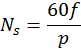# Wound-rotor synchronous motor

Wound-rotor synchronous motor is a synchronous electric motor, the rotor of which is made with a field winding.

## Construction of wound-rotor synchronous motor

Wound-rotor synchronous motor like any rotating electric motor, consists of a rotor and a stator. The stator is the fixed part. The rotor is the rotating part. The stator usually has a standard three-phase winding, and the rotor is made with a field winding. The field winding is connected to slip rings to which power is supplied through brushes.

Wound-rotor synchronous motor (brushes not shown)

## Working principle

The constant speed of a synchronous motor is achieved by the interaction between a constant and rotating magnetic field. The rotor of the synchronous motor creates a constant magnetic field, and the stator - a rotating magnetic field.

The operation of the synchronous motor is based on the interaction of the rotating magnetic field of the stator and the constant magnetic field of the rotor

### Stator: rotating magnetic field

A three-phase AC supply is applied to the windings of the stator coils. The result is a rotating magnetic field that rotates at a speed proportional to the frequency of the supply voltage. Read more about how a rotating magnetic field is generated by a three-phase supply voltage in the article "Three phase induction motor".

The interaction between rotating (stator) and constant (rotor) magnetic fields

### Rotor: constant magnetic field

The rotor winding is excited by a direct current source through slip rings. The magnetic field created around the rotor excited by direct current is shown below. Obviously, the rotor behaves like a permanent magnet, since it has the same magnetic field (as an alternative, you can imagine that the rotor is made of permanent magnets). Consider the interaction of the rotor and the rotating magnetic field. Suppose you give the rotor an initial rotation in the same direction as the rotating magnetic field. The opposite poles of the rotating magnetic field and the rotor will be attracted to each other and they will get locked with the help of magnetic forces. This means that the rotor will rotate at the same speed as the rotating magnetic field, that is, the rotor will rotate at a synchronous speed.

Magnetic fields of the rotor and stator are locked to each other

## Synchronous speed

The speed at which the magnetic field rotates can be calculated by the following equation:,

• where Ns – rotation frequency of the magnetic field, rpm,
• f – stator current frequency, Hz,
• p – number of pole pairs.

This means that the speed of the synchronous motor can be accurately controlled by varying the frequency of the supply current. Thus, these electric motors are suitable for high precision applications.

## Direct-on-line starting of synchronous motor

### Why are synchronous motors not self-starting from the electrical grid?

If the rotor does not have an initial rotation, the situation is different from that described above. The north pole of the magnetic field of the rotor will be attracted to the south pole of the rotating magnetic field and will begin to move in the same direction. But since the rotor has a certain moment of inertia, its starting speed will be very low. During this time, the south pole of the rotating magnetic field will be replaced by the north pole. In this way, repulsive forces will appear. As a result, the rotor will begin to rotate in the opposite direction, and will not be started.

### Damper winding - the direct start of a synchronous motor from an electrical grid

In order to realize a self-starting of a synchronous motor without a control system, a “squirrel cage” is placed between the tips of the rotor, which is also called a damper winding. When starting the motor, the rotor coils are not excited. Under the action of a rotating magnetic field, a current is induced in the turns of the "squirrel cage" and the rotor starts to rotate just like induction motors are started.

When the rotor reaches its maximum speed, power is supplied to the field winding of the rotor. As a result, the rotor poles interlock with the poles of the rotating magnetic field and the rotor begins to rotate at a synchronous speed. When the rotor rotates at synchronous speed, the relative motion between the squirrel cage and the rotating magnetic field is zero. This means that there is no current in short-circuited turns, and therefore the "squirrel cage" does not affect the synchronous operation of the electric motor.

## Out of synchronism

Synchronous electric motors have a constant speed independent of the load (provided that the load does not exceed the maximum permissible). If the load torque is greater than the torque created by the electric motor itself, then it will come out of synchronism and stop. Low supply voltage and low excitation voltage can also be the cause of going out of sync.

## Synchronous condenser

Synchronous motors can also be used to improve the power factor of a system. When the single purpose of using synchronous motors is to improve the power factor, they are called synchronous compensators. In this case, the motor shaft is not connected to the mechanical load and rotates freely.